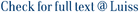Verification theorems are key results to successfully employ the dynamic programming approach to optimal control problems. In this paper, we introduce a new method to prove verification theorems for infinite dimensional stochastic optimal control problems. The method applies in the case of additively controlled Ornstein–Uhlenbeck processes, when the associated Hamilton–Jacobi–Bellman (HJB) equation admits a mild solution (in the sense of [J. Differential Equations 262 (2017) 3343–3389]). The main methodological novelty of our result relies on the fact that it is not needed to prove, as in previous literature (see, e.g., [Comm. Partial Differential Equations 20 (1995) 775–826]), that the mild solution is a strong solution, that is, a suitable limit of classical solutions of approximating HJB equations. To achieve the goal, we prove a new type of Dynkin formula, which is the key tool for the proof of our main result.

Verification theorems for stochastic optimal control problems in Hilbert spaces by means of a generalized Dynkin formula / Federico, Salvatore; Gozzi, Fausto. - In: THE ANNALS OF APPLIED PROBABILITY. - ISSN 1050-5164. - 28:6(2018), pp. 3558-3599.

### Verification theorems for stochastic optimal control problems in Hilbert spaces by means of a generalized Dynkin formula

#### Abstract

Verification theorems are key results to successfully employ the dynamic programming approach to optimal control problems. In this paper, we introduce a new method to prove verification theorems for infinite dimensional stochastic optimal control problems. The method applies in the case of additively controlled Ornstein–Uhlenbeck processes, when the associated Hamilton–Jacobi–Bellman (HJB) equation admits a mild solution (in the sense of [J. Differential Equations 262 (2017) 3343–3389]). The main methodological novelty of our result relies on the fact that it is not needed to prove, as in previous literature (see, e.g., [Comm. Partial Differential Equations 20 (1995) 775–826]), that the mild solution is a strong solution, that is, a suitable limit of classical solutions of approximating HJB equations. To achieve the goal, we prove a new type of Dynkin formula, which is the key tool for the proof of our main result.
##### Scheda breve Scheda completa Scheda completa (DC)File in questo prodotto:
File
euclid.aoap.1538985629.pdf

Solo gestori archivio

Descrizione: Articolo Principale
Tipologia: Versione dell'editore
Licenza: Tutti i diritti riservati
Dimensione 389.39 kB
Utilizza questo identificativo per citare o creare un link a questo documento: `http://hdl.handle.net/11385/183321`
•2
•2